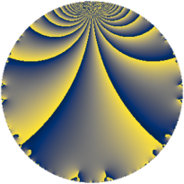# Properties

 Label 3822.2.a.iLevel $3822$ Weight $2$ Character orbit 3822.a Self dual yes Analytic conductor $30.519$ Analytic rank $1$ Dimension $1$ CM no Inner twists $1$

# Related objects

Show commands: Magma / PariGP / SageMath

## Newspace parameters

comment: Compute space of new eigenforms

[N,k,chi] = [3822,2,Mod(1,3822)]

mf = mfinit([N,k,chi],0)

lf = mfeigenbasis(mf)

from sage.modular.dirichlet import DirichletCharacter

H = DirichletGroup(3822, base_ring=CyclotomicField(2))

chi = DirichletCharacter(H, H._module([0, 0, 0]))

N = Newforms(chi, 2, names="a")

//Please install CHIMP (https://github.com/edgarcosta/CHIMP) if you want to run this code

chi := DirichletCharacter("3822.1");

S:= CuspForms(chi, 2);

N := Newforms(S);

 Level: $$N$$ $$=$$ $$3822 = 2 \cdot 3 \cdot 7^{2} \cdot 13$$ Weight: $$k$$ $$=$$ $$2$$ Character orbit: $$[\chi]$$ $$=$$ 3822.a (trivial)

## Newform invariants

comment: select newform

sage: f = N # Warning: the index may be different

gp: f = lf \\ Warning: the index may be different

 Self dual: yes Analytic conductor: $$30.5188236525$$ Analytic rank: $$1$$ Dimension: $$1$$ Coefficient field: $$\mathbb{Q}$$ Coefficient ring: $$\mathbb{Z}$$ Coefficient ring index: $$1$$ Twist minimal: no (minimal twist has level 546) Fricke sign: $$1$$ Sato-Tate group: $\mathrm{SU}(2)$

## $q$-expansion

comment: q-expansion

sage: f.q_expansion() # note that sage often uses an isomorphic number field

gp: mfcoefs(f, 20)

 $$f(q)$$ $$=$$ $$q - q^{2} - q^{3} + q^{4} + 2 q^{5} + q^{6} - q^{8} + q^{9}+O(q^{10})$$ q - q^2 - q^3 + q^4 + 2 * q^5 + q^6 - q^8 + q^9 $$q - q^{2} - q^{3} + q^{4} + 2 q^{5} + q^{6} - q^{8} + q^{9} - 2 q^{10} + 3 q^{11} - q^{12} + q^{13} - 2 q^{15} + q^{16} - 5 q^{17} - q^{18} - q^{19} + 2 q^{20} - 3 q^{22} + q^{24} - q^{25} - q^{26} - q^{27} - q^{29} + 2 q^{30} - 4 q^{31} - q^{32} - 3 q^{33} + 5 q^{34} + q^{36} - 2 q^{37} + q^{38} - q^{39} - 2 q^{40} - 10 q^{41} - 10 q^{43} + 3 q^{44} + 2 q^{45} + q^{47} - q^{48} + q^{50} + 5 q^{51} + q^{52} - 3 q^{53} + q^{54} + 6 q^{55} + q^{57} + q^{58} - 3 q^{59} - 2 q^{60} + 5 q^{61} + 4 q^{62} + q^{64} + 2 q^{65} + 3 q^{66} + 5 q^{67} - 5 q^{68} - q^{71} - q^{72} - 12 q^{73} + 2 q^{74} + q^{75} - q^{76} + q^{78} - 6 q^{79} + 2 q^{80} + q^{81} + 10 q^{82} - 16 q^{83} - 10 q^{85} + 10 q^{86} + q^{87} - 3 q^{88} + 14 q^{89} - 2 q^{90} + 4 q^{93} - q^{94} - 2 q^{95} + q^{96} - 4 q^{97} + 3 q^{99}+O(q^{100})$$ q - q^2 - q^3 + q^4 + 2 * q^5 + q^6 - q^8 + q^9 - 2 * q^10 + 3 * q^11 - q^12 + q^13 - 2 * q^15 + q^16 - 5 * q^17 - q^18 - q^19 + 2 * q^20 - 3 * q^22 + q^24 - q^25 - q^26 - q^27 - q^29 + 2 * q^30 - 4 * q^31 - q^32 - 3 * q^33 + 5 * q^34 + q^36 - 2 * q^37 + q^38 - q^39 - 2 * q^40 - 10 * q^41 - 10 * q^43 + 3 * q^44 + 2 * q^45 + q^47 - q^48 + q^50 + 5 * q^51 + q^52 - 3 * q^53 + q^54 + 6 * q^55 + q^57 + q^58 - 3 * q^59 - 2 * q^60 + 5 * q^61 + 4 * q^62 + q^64 + 2 * q^65 + 3 * q^66 + 5 * q^67 - 5 * q^68 - q^71 - q^72 - 12 * q^73 + 2 * q^74 + q^75 - q^76 + q^78 - 6 * q^79 + 2 * q^80 + q^81 + 10 * q^82 - 16 * q^83 - 10 * q^85 + 10 * q^86 + q^87 - 3 * q^88 + 14 * q^89 - 2 * q^90 + 4 * q^93 - q^94 - 2 * q^95 + q^96 - 4 * q^97 + 3 * q^99

## Embeddings

For each embedding $$\iota_m$$ of the coefficient field, the values $$\iota_m(a_n)$$ are shown below.

For more information on an embedded modular form you can click on its label.

comment: embeddings in the coefficient field

gp: mfembed(f)

Label $$\iota_m(\nu)$$ $$a_{2}$$ $$a_{3}$$ $$a_{4}$$ $$a_{5}$$ $$a_{6}$$ $$a_{7}$$ $$a_{8}$$ $$a_{9}$$ $$a_{10}$$
1.1
 0
−1.00000 −1.00000 1.00000 2.00000 1.00000 0 −1.00000 1.00000 −2.00000
 $$n$$: e.g. 2-40 or 990-1000 Significant digits: Format: Complex embeddings Normalized embeddings Satake parameters Satake angles

## Atkin-Lehner signs

$$p$$ Sign
$$2$$ $$1$$
$$3$$ $$1$$
$$7$$ $$-1$$
$$13$$ $$-1$$

## Inner twists

This newform does not admit any (nontrivial) inner twists.

## Twists

By twisting character orbit
Char Parity Ord Mult Type Twist Min Dim
1.a even 1 1 trivial 3822.2.a.i 1
7.b odd 2 1 3822.2.a.k 1
7.d odd 6 2 546.2.i.e 2
21.g even 6 2 1638.2.j.a 2

By twisted newform orbit
Twist Min Dim Char Parity Ord Mult Type
546.2.i.e 2 7.d odd 6 2
1638.2.j.a 2 21.g even 6 2
3822.2.a.i 1 1.a even 1 1 trivial
3822.2.a.k 1 7.b odd 2 1

## Hecke kernels

This newform subspace can be constructed as the intersection of the kernels of the following linear operators acting on $$S_{2}^{\mathrm{new}}(\Gamma_0(3822))$$:

 $$T_{5} - 2$$ T5 - 2 $$T_{11} - 3$$ T11 - 3 $$T_{17} + 5$$ T17 + 5 $$T_{29} + 1$$ T29 + 1

## Hecke characteristic polynomials

$p$ $F_p(T)$
$2$ $$T + 1$$
$3$ $$T + 1$$
$5$ $$T - 2$$
$7$ $$T$$
$11$ $$T - 3$$
$13$ $$T - 1$$
$17$ $$T + 5$$
$19$ $$T + 1$$
$23$ $$T$$
$29$ $$T + 1$$
$31$ $$T + 4$$
$37$ $$T + 2$$
$41$ $$T + 10$$
$43$ $$T + 10$$
$47$ $$T - 1$$
$53$ $$T + 3$$
$59$ $$T + 3$$
$61$ $$T - 5$$
$67$ $$T - 5$$
$71$ $$T + 1$$
$73$ $$T + 12$$
$79$ $$T + 6$$
$83$ $$T + 16$$
$89$ $$T - 14$$
$97$ $$T + 4$$